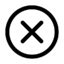MAY 1, 2009MYSTERY

A DRINK FOR CROW

Once there was a crow who had grown so thirsty he could barely crow.  He flew down to a big pitcher where he had gotten a drink of water the day before, but there was only a little bit of water remaining at the bottom.  He tried and tried to reach it with his beak, but the pitcher was too deep and his beck was too short.  But just as he was about to give up, he knew what to do.  He flew back and forth from the garden to the pitcher until his was able to drink easily from the pitcher while sitting on its edge.
WHAT DID THE CROW DO???

SEE NEXT MONTH FOR THE ANSWER.FROM LAST MONTH

AMAZING  NUMBERS

 11.111.111 x 111.111.111 = 12.345.678.987.654.321 Multiply of 37 by multiplies of 3: 3 x 37 = 111 6 x 37 = 222 9 x 37 = 333 12 x 37 = 444 15 x 37 = 555 18 x 37 = 666 21 x 37 = 777 24 x 37 = 888 27 x 37 = 999 Trapeze: 1 x 9 + 2 = 11 12 x 9 + 3 = 111 123 x 9 + 4 = 1111 1234 x 9 + 5 = 11111 12345 x 9 + 6 = 111111 123456 x 9 + 7 = 1111111 1234567 x 9 + 8 = 11111111 12345678 x 9 + 9 = 111111111 Trapeze #2: 1 x 8 + 1 = 9 12 x 8 + 2 = 98 123 x 8 + 3 = 987 1234 x 8 + 4 = 9876 12345 x 8 + 5 = 98765 123456 x 8 + 6 = 987654 1234567 x 8 + 7 = 9876543 12345678 x 8 + 8 = 98765432 123456789 x 8 + 9 = 987654321 0 x 9 + 8 = 8 9 x 9 + 7 = 88 98 x 9 + 6 = 888 987 x 9 + 5 = 8888 9876 x 9 + 4 = 88888 98765 x 9 + 3 = 888888 987654 x 9 + 2 = 8888888 9876543 x 9 + 1 = 88888888 98765432 x 9 + 0 = 888888888 987654321 x 9 - 1 = 8888888888 9876543210 x 9 - 2 = 88888888888 123456789 x 0.9 = 11111111 123456789 x 1.8 = 22222222 123456789 x 2.7 = 33333333 123456789 x 3.6 = 44444444 123456789 x 4.5 = 55555555 123456789 x 5.4 = 66666666 123456789 x 6.3 = 77777777 123456789 x 7.2 = 88888888 123456789 x 8.1 = 99999999CLICK BELOW FOR HUMMING HORSES

Don't forget to click BACKYOU CAN HAVE YOUR OWN PRIVATE EYE WHICH YOU CONTROL WITH YOUR CURSOR.  JUST CLICK THE THE LINK BELOW   EYEBALL DON'T FORGET to CLICK BACK
 HOME NEWS HUMOR BOOKS COUNTRY CORNER LETTERS ARCHIVES